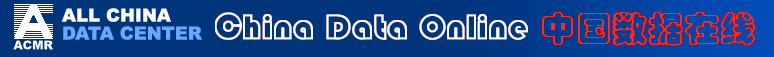Welcome Guest , your IP is: 3.215.79.204       Today is: 2022/6/27 EST USALocation: All China Data   | Support
 07. PRODUCTION AND CONSUMPTION OF ENERGY

 Indicator Explanation Total Energy Production refers to the total production of primary energy by all energy producing enterprises in the country in a given period of time. It is a comprehensive indicator to show the capacity, scale, composition and development of energy production of the country. The production of primary energy includes that of coal, crude oil, natural gas, hydro-power and electricity generated by nuclear energy and other means such as wind power and geothermal power. However, it excludes the production of fuels of low calorific value, bio-energy, solar energy and the secondary energy converted from the primary energy. Total Domestic Energy Consumption refers to the total consumption of energy of various kinds by material production sectors, non material production sectors and households in the country in a given period of time. It is a comprehensive indicator to show the scale, composition and development of energy consumption. The total energy consumption includes that of coal, crude oil and their products, natural gas and electricity, However, it excludes the consumption of fuel of low calorific value, bio-energy and solar energy. Total domestic energy consumption can be divided into three parts: (1)Final Energy Consumption: It refers to the total energy consumption by material production sectors, non material production sectors and households in the country (region) in a given period of time, but excludes the consumption in conversion of the primary energy into the secondary energy and the loss in the process of energy conversion. (2)Loss During the Process of Energy Conversion: It refers to the total input of various kinds of energy for conversion, minus the total output of various kinds of energy in the country in a given period of time. It is an indicator to show the loss that occurs during the process of energy conversion. (3)Loss: It refers to the total of the loss of energy during the course of energy transport, distribution and storage and the loss caused by any objective reason in a given period of time. The loss of various kinds of gas due to gas discharges and stocktaking is excluded. Elasticity Ratio of Energy Production is an indicator to show the relationship between the growth rate of energy production and the growth rate of the national economy. The formula is: Elasticity Ratio of Energy Production = Average Annual Growth Rate of Energy Production Average Annual Growth Rate of National Economy The average annual growth rate of the national economy can be shown by the gross national product, gross domestic product and other indicators, depending upon the purposes or needs. The gross domestic product is used in calculation of the ratio in this chapter. Elasticity Ratio of Electricity Production is an indicator to show the relationship between the growth rate of electricity production and the growth rate of the national economy. Generally speaking, the growth rate of electricity production should be higher than that of the national economy. Its formula is: Elasticity Ratio of Electricity Production = Average Annual Growth Rate of Electricity Production Average Annual Growth Rate of National Economy Elasticity Ratio of Energy Consumption is an indicator to show the relationship between the growth rate of energy consumption and the growth rate of the national economy. The formula is: Elasticity Ratio of Energy Consumption = Average Annual Growth Rate of Energy Consumption Average Annual Growth Rate of National Economy Elasticity Ratio of Electricity Consumption is an indicator to show the relationship between the growth rate of electricity consumption and the growth rate of the national economy. The formula is: Elasticity Ratio of Electricity Consumption = Average Annual Growth Rate of Electricity Average Annual Growth Rate of National Economy Efficiency of Energy Processing and Conversion refers to the ratio of the total output of energy products of various kinds after processing and conversion and the total input of energy of various kinds for processing and conversion in the same reference period. It is an important indicator to show the current conditions of energy processing and conversion equipment, production technique and management. The formula is: Efficiency of Energy Processing & Conversion = Output of Energy After Processing & Conversion / Input of Energy for Processing & ConversionˇÁ100%